Skip to main content

Volume 20 Supplement 13

# An efficient simulated annealing algorithm for the RNA secondary structure prediction with Pseudoknots

## Abstract

### Background

RNA pseudoknot structures play an important role in biological processes. However, existing RNA secondary structure prediction algorithms cannot predict the pseudoknot structure efficiently. Although random matching can improve the number of base pairs, these non-consecutive base pairs cannot make contributions to reduce the free energy.

### Result

In order to improve the efficiency of searching procedure, our algorithm take consecutive base pairs as the basic components. Firstly, our algorithm calculates and archive all the consecutive base pairs in triplet data structure, if the number of consecutive base pairs is greater than given minimum stem length. Secondly, the annealing schedule is adapted to select the optimal solution that has minimum free energy. Finally, the proposed algorithm is evaluated with the real instances in PseudoBase.

### Conclusion

The experimental results have been demonstrated to provide a competitive and oftentimes better performance when compared against some chosen state-of-the-art RNA structure prediction algorithms.

## Background

RNA is a linear molecular compound formed by polymerization of ribonucleotides with phosphodiester bonds, the ribonucleotides are composed of phosphoric acid, ribose and bases. The RNA sequence consists of Adenine (A), Uracil (U), Guanine (G) and Cytosine (C), the four-base arrangement allows RNA to have a variety of functions that can play great role in genetic coding, translation, regulation, and gene expression. The search for the secondary structure of RNA sequence has been widely used as the first step to understand biological functions .

Pseudoknot is a special RNA secondary structure that is found in many important biologically molecules [2, 3], it usually contains not well-nested base pairs. These non-nested base pairs make the presence of pseudoknots in RNA sequences more difficult to be predicted by dynamic programming, which use a recursive scoring system to identify paired stems. The general problem of predicting minimum free energy (MFE) structures with pseudoknots is NP-complete problem . In general, researchers apply the principle of MFE to evaluate RNA secondary structure. When the RNA sequence is freely folded in space to form the secondary structure of MFE under fixed experimental conditions, the change is stopped, meanwhile, the stable state of the RNA sequence is formed. For the calculation of the free energy of RNA secondary structure, the stem energy is defined as a negative, the energy of loop is defined as a positive, and the free single strand does not participate. Deng found that the molecular free energy is related to a single complementary base pair, but adjacent base pairs also affect the free energy calculation of the molecule . In the secondary structure prediction, if the free energy calculation of each part does not affect each other, the free energy of the entire structure is accumulated form the energy of each part, and the calculation principle is shown in Eq. (1).

$$\varDelta G=\sum \varDelta {G}_S+\sum \varDelta {G}_H+\sum \varDelta {G}_I+\sum \varDelta {G}_B+\sum \varDelta {G}_M+\sum \varDelta {G}_P+\varDelta \delta$$
(1)

In the above formula, ΔGS means the stem free energy; ΔGH, ΔGI, ΔGB, and ΔGM represent the free energy of hairpin, internal, bulged, and multi-branch loop, respectively; ΔGP represent the pseudoknot free energy, which is generally split into loop for calculation to simplify the calculation process; Δδ is a threshold set to balance the error during the experiment process. After the RNA secondary structure is calculated in the Eq. (1), researcher can objectively evaluate whether the current structure is stable by numerical changes.

At present, existing algorithms for the prediction of RNA secondary structure with pseudoknots can be classified into two categories. The first category is dynamic programming (DP) based approaches. DP is the initial computational approach used to predict RNA structure . The idea of dynamic programming is to divide a complex problem into many simple sub-problems to facilitate their treatment . Combining the DP idea with the principle of MFE, researchers have proposed many RNA secondary structure prediction algorithms. Rivas and Eddy  proposed pknots-RE algorithm that can predict RNA sequence with pseudoknot structure. Dirks and Pierce  proposed NUPACK algorithm which calculate a series of recursion probabilities that can be used to compute base-pairing probabilities with or without pseudoknots. However, these algorithms are very time-consuming to predict long-chain sequence, and its maximum predictive sequence length cannot exceed 150.

The second category is Heuristic based approaches, which can handle long RNA sequences and obtain high quality feasible solution efficiently . Ren et al.  proposed HotKnots to build up candidate secondary structures by adding substructures one by one to partially formed structures. Zuker et al.  and Turner et al.  integrate thermodynamic model into their algorithms to search for secondary structure with minimal free energy. SARNA-predict-pk  algorithm is an extended version of SARNA-Predict  which predicts RNA secondary structures with pseudoknots. This algorithm employs a new thermodynamic model that was described by Rastegari and Condon  and implemented in the HotKnots software. The model can be used to evaluate RNA sequences with pseudoknots. IPknot  algorithm proposed a computational method for predicting RNA secondary structures with pseudoknots based on maximizing the expected accuracy of a predicted structure. Iterative HFold  takes as input a pseudoknot-free structure, and produces a possibly pseudoknotted structure whose energy is at least as low as that of any (density-2) pseudoknotted structure containing the input structure. It leverages strengths of earlier methods, namely the fast running time of HFold, a method that is based on the hierarchical folding hypothesis and the energy parameters of HotKnots V2.0. Fatmi et al.  proposed a new algorithm that combines between the Greedy Randomized Adaptive Search Procedure (GRASP) and the Genetic Algorithm (GA) principle. This method repeats a process consisting of two phases: the construction phase and the local search phase. During the construction phase, a list of feasible solutions is iteratively constructed. The local search phase comes with the wake of the construction step; it aims to improve the solution obtained from the first phase by launching a local search to find the local optimum solution.

In this paper, a novel efficient simulated annealing (SA) algorithm is proposed to predict RNA secondary structure with pseudoknot. Firstly, an efficient base pairing method is designed, which is based on the minimum stem length and the minimum loop length, and a completed conflict resolution is provided for the conflicting bases; Then a simple and effective fitness function is proposed, and the number of stem and the total number of base pairs of the RNA sequence is used as metrics for evaluating the secondary structure of RNA; Finally, the annealing schedule is selected to systematically decrease the temperature as the algorithm proceeds, the final solution is the structure with MFE. In this paper, eighteen test sequences are randomly selected from the PseudoBase , and the results are compared with other leading prediction algorithms such as HotKnots , IPknot , TT2NE , CombFold , RnaStructure , CyloFold  and RNAflod  which shows, the effectiveness of our algorithm.

## Methods

The RNA secondary structure folds itself by forming hydrogen bonds between G-C, A-U, and G-U. Therefore, the prediction of all hydrogen connections among the primary structure of the sequence become the first in predicting RNA secondary structure. Many components can be identified in the secondary structure, such as stem, hairpin loop, multi-branched loop or multi-loops, bulge loop, internal loop, and pseudoknot, as shown in Fig. 1.Fig. 1

### Definition

For a given RNA sequence X = 5′-x1x2…, xi, … xn-3′ of length n, i is defined as the initial index of the current base and Y(X) is the mapping string of consecutive complementary base pairs of X, Y(X) = (y1, y2, …, yi, …, yn), yi is assigned to be j, if base xi bond with base xj, as shown in Eq. 2.

$${y}_i=\Big\{\begin{array}{c}j,\mathrm{if}\;{x}_i\;\mathrm{paired}\kern0.17em \mathrm{with}\;{x}_j\\ {}i,\mathrm{else}\kern6em \end{array}}$$
(2)

As shown in Fig. 2, when the base is paired, the sequence numbers of the paired bases are exchanged and stored in Y(X), then Y(X) = (1, 14, 13, 12, 5, 6, 7, 8, 9, 10, 11, 4, 3, 2, 15). Each mapping string Y(X) is a candidate solution, the solution with MFE is the optimal solution, which is the most stable secondary structure.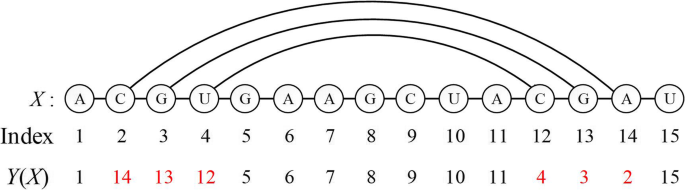Fig. 2

In order to better simulate the folding process of RNA secondary structure in the program, we define each part of the RNA secondary structure as follows:

Definition 1: X = 5′-x1x2xn-3′, xi {A, U, G, C}, Sequence X is called an RNA sequence of length n.

Definition 2 (stem): xixi + 1xi + k-1 and xj-k + 1xj-1xj is two sub-segments in sequence X, (xi, xj) W = {(A, U), (U, A), (G, C), (C, G), (G, U), (U, G)}, 1 ≤ i < jn, j − i≥ 3, then the structure of consecutive base pairing by {(xi, xj), (xi + 1, xj-1),…, (xi + k-1, xj-1)} is called the stem of length k (k ≥ 2). To simplify calculations, stem can be expressed as a mi = (i, j, k), where parameters i and j are the index of beginning base and ending base, and parameter k is the length of this stem.

Definition 3 (hairpin Loop): There must be at least MinLoop (MinLoop ≥ 3) unpaired bases in any hairpin loop structure.

Definition 4 (consecutive complementary base paired set): The complete RNA secondary structure of a sequence X is called a consecutive complementary base pair set, recorded as M(X), M(X) = (m1, m2,…, mi, …,mn). Each mi represents a stem, according to the above definition, any mi can be recorded as (i, j, k). In the sequence X, the secondary structure formed by the pairing of M(X) is represented by Y(X).

Definition 5 (pseudoknot): xp, xq, xr, xs, X, (xp, xq), (xr, xs) W, and the number of four bases in X satisfies 1 ≤ p < r < q < sn or 1 ≤ r < p < s < qn, then the structure formed by these two base pairs is called a pseudoknot structure, as shown in Fig. 3.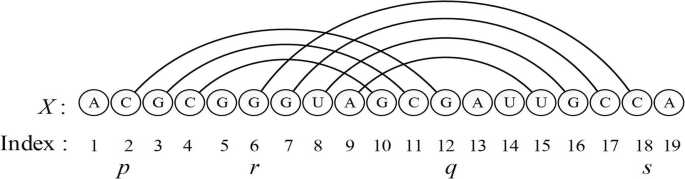Fig. 3

According to the above definition, the secondary structure prediction problem with pseudoknot can be converted to find the number of stems in all possible stem of the X sequence. These stems are so unique that secondary structure formed by their base complementarity has MFE state. Thus, an efficient Prediction algorithm of RNA secondary structure with pseudoknot based on SA (PRSA) is proposed.

### Set of K consecutive base pairs

Since single base pairs cannot contribute to the reduction of free energy, the PRSA algorithm considers consecutive base pairs. In order to find all the stem structures, we defined the minimum stem length (MinStem ≥ 2) and the minimum loop length (MinLoop ≥ 3) parameters, as shown in Fig. 4.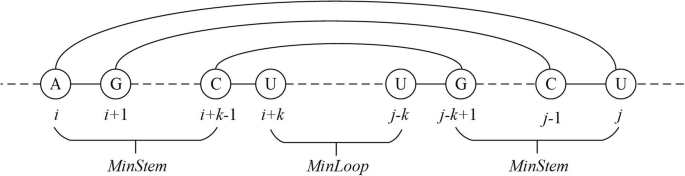Fig. 4

After initially setting the parameters MinStem and MinLoop, all the reasonable mi can be calculated. Parameters i, j and k need to satisfy the following three constraints:

$$1\le i\le n-2\ast MinStem- MinLoop+1$$
(3)
$$i+2\ast MinStem+ MinLoop-1\le j\le n$$
(4)
$$MinStem\le k\le \frac{j-i- MinLoop+1}{2}$$
(5)

For example, Mengo_PKB is an RNA molecule from the PseudoBase, whose sequence is 5 − ACGUGAAGGCUACGAUAGUGCCAG − 3. Let MinStem and MinLoop be 3, all possible triplets (i, j, k) are (2,14,3), (2,14,4), (2,20,3), (3,13,3), (3,21,3), (8,22,3), (9,19,4), (10,18,3), (11,20,3). The pseudo code of calculation consecutive base pairs is shown as Algorithm 1.

But in all base pairs, the same position of bases may have different consecutive base pair numbers, we need to merge these same positions. Like the above Mengo_PKB sequence, the set of base pairs after the merge is (2, 14, (3, 4)), (2, 20, (3)), (3, 13, (3)), (3, 21, (3)), (8, 22, (3)), (9, 19, (3, 4)), (10, 18, (3)), (11, 20, (3)). The pseudo code that saves the merged result to the K consecutive base pair set is shown in Algorithm 2.

As known that most predicted algorithms require more effort to calculate the MFE structure after calculating the free energy of the current prediction, which makes their algorithm converge very slowly. A pool of candidate structures is generated by constructing a set of K consecutive base pairs, which makes the PRSA algorithm converge faster than other prediction algorithms. This also makes each iteration more valuable because each iteration generates a new structure from the candidate pool.

### Neighbor state and its conflict

When the secondary structure prediction is performed on any of the RNA molecules, the PRSA algorithm would first calculate the K consecutive base pair set by parameter preprocessing, and then generate a neighbor state through a random function in the simulated annealing algorithm.

Taking the TMEV molecule as an example, after the preprocessing process of the upper section ‘Set of K consecutive base pairs’, a K consecutive base pairs set of TMEV molecules is obtained, as shown in Fig. 5.Fig. 5

Divided according to the base start position and end position of stem, this set contains 13 elements. Since the base start and end positions of the stem are the same, different stem lengths may exist, so the algorithm determines one stem by generating two random numbers. The first random number is between 1 and 13, and the second random number is related to its corresponding set of K consecutive base pairs.

For example, take two random values as 10 and 1, respectively. At this time, m1 = (9, 19, 3), a local RNA secondary structure is formed. In order to be recorded in the programming, this section of the algorithm has been processed in 4 steps:

(1) The paired base numbers are exchanged as shown in Fig. 6, m1 is added to the consecutive base pair set M(X), at this time M(X) = {m1 = (9, 19, 3)}, and the secondary structure corresponding to M(X) is represented by Y1(X).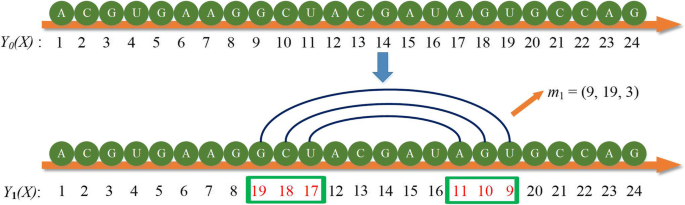Fig. 6

(2) A randomly generated mi that may conflict with elements in the set M(X). When the algorithm program performs the next iteration of the loop, a new stem m2 = (2, 20, 3) is generated. At this time, a base pairing conflict occurs, that is, the bases originally numbered 18 and 19 have been paired with the bases at other positions, and the base complementary pairing conflicts are shown in Fig. 7.Fig. 7

(3) If there is a conflict, the position number of the conflicting base is exchanged again to remove the conflict, and the m1 in the M(X) is updated, and the schematic diagram of removing the base pairing conflict is shown in Fig. 8. The M(X) is updated to {m1 = (11, 17, 1)} after removal.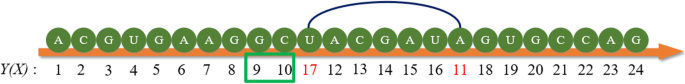Fig. 8

(4) Determine whether the updated mi meets the constraint. If it does not, remove it; if it does, it will not be considered. When the constraint is initialized, the algorithm program sets the minimum length of the stem to be no smaller than MinStem. Assume that the initial value of MinStem is 3, therefore, the remaining pairing mode of m1 needs to be removed, and the element is deleted from M(X), and M(X) is an empty set. The operation process is shown in Fig. 9.Fig. 9

After the conflicts and constraints are resolved, the base pairing is performed in the new stem and added to M(X), as shown in Fig. 10. At this time, M(X) = {m2 = (2, 20, 3)}, the secondary structure corresponding to M(X) is represented by Y2(X), and Y2(X) is the neighbor state of Y1(X).Fig. 10

### Fitness function

For most MFE based RNA secondary structure prediction algorithm, the complex thermodynamic model is often used to evaluate candidate solutions . However, there is no useful information to guide the candidate solution to find lower neighbor energy state. Consequently, the convergence of these MFE based prediction algorithms is very slow. Actually, only the consecutive base pairs stem ∆GS provide negative free energy which contributes to the reduction of free energy. The stability of RNA sequence can also be approximately evaluated by consecutive base pairs stem.

Where Group is the number of stems of the secondary structure of the RNA sequence, TP is the sum of the number of all base pairs in the sequence, TP divided by Group is the average number of base pairs (AP), PG is the predicted number of pseudoknots by the algorithm, MG is the expected number of pseudoknots, and k is the length of the stem. The evaluation function for random candidate M(X) can be seen in the following Equation:

$$F\left(M(X)\right)=\Big\{\begin{array}{cc} TP\times A{P}^2,& PG\le MG\\ {} TP\times A{P}^2\times \frac{Group- PG}{Group},& PG> MG\end{array}}$$
(6)
$$TP=\sum \limits_{i=1}^n{m}_i.k$$
(7)
$$AP=\frac{TP}{Group}$$
(8)

The two structures of the BCRV1 molecule are evaluated using the custom fitness function,

M1(X) = {m1 = (5,47,6), m2 = (14,80,6), m3 = (20,38,5), m4 = (26,98,7), m5 = (53,74,9)}, as shown in Fig. 11a; M2(X) = {m1 = (4,48,8), m2 = (19,39,6), m3 = (26,98,7), m4 = (52,75,10)}, as shown in Fig. 11b. We produce the images of RNA structure with jViz. Rna .Fig. 11

After evaluation, the calculated data of the secondary structure of BCRV1 molecule are shown in Table 1. According to the fitness function values of the two structures, it indicates that M2 is better than M1.

### Overall algorithm

The PRSA algorithm initializes the parameters to determine the constraints of the RNA sequence, thereby calculating a set of K consecutive base pairs. According to this set, the neighbor state is randomly generated, and the custom fitness function is adopted to evaluate the quality of the current solution (CurrentPairs) and the previous generation solution (MaxPairs). If the CurrentPairs performs better, it would replace the MaxPairs directly. Otherwise, it will determine whether to accept the new pairing structure based on probability from Boltzmann distribution. The final predicted solution structure is stored in MaxPairs, which has MFE and includes pseudoknot. The pseudo-code of the overall algorithm is shown in Algorithm 3.

## Result

In section ‘method’, Predicting RNA secondary structures with pseudoknots is implemented using the PRSA algorithm. In the following, we first present the datasets, the exiting methods and accuracy measures we use for the evaluation of the algorithm, then the prediction performance of the PRSA algorithm is demonstrated by comparative experiments.

### Data sets

The eighteen benchmark instances from PseudoBase were used to test the proposed method. The characteristic of each sequence is shown in Table 2. The second column is the Abbreviation of the RNA sequence, the third column is the RNA PKB number, the fourth column is the RNA type, the fifth column is the sequence length and the last column is the number of base pairs in the known structure. The predicted structure should be similar to the base pairs of the known structure.

### Accuracy measures

The prediction accuracy is calculated by comparing the predicted structure with the known structure. In order to assess the quality of the results produced, three evaluation criteria were used: sensitivity (SN%), specificity (SP%) and F-measure(%) . The evaluation criteria are as follows:

$$SN= TP\div \left( TP+ FN\right)$$
(9)
$$SP= TP\div \left( TP+ FP\right)$$
(10)
$$F- measure=2\ast SP\ast SN\div \left( SN+ SP\right)$$
(11)

Where TP represents the number of correctly predicted base pairs; FP represents the number of incorrectly predicted base pairs; FN represents the number of unpredicted base pairs compared with the known structure. When the prediction results are accurate, both SN and SP should be close to 100%.

### Comparison with existing methods

To better reflect the accuracy of the algorithm proposed in this paper, the computational results of the PRSA algorithm are compared with seven state-of-the-art algorithms, including HotKnots , IPknot , TT2NE , CombFold , RnaStructure , CyloFold  and RNAflod . Among these algorithms, the HotKnots algorithm and the IPknot algorithm use heuristic ideas to predict the secondary structure. The names of the seven algorithms and the website links to the algorithm-based Web sites are listed in Table 3.

### Overall results

The comparisons of the proposed method with the other methods are shown in Tables 4, 5 and 6. If the value in the table is “#”, it means that the algorithm does not support the prediction of the length of the sequence, such as TT2NE. The results of the proposed method and the compared methods are all run 10 times for each sequence.

From Table 4, in terms of sensitivity, the proposed method provides the best results in nineteen sequences, of which 9 sequences predict 100%. In addition, there are 3 sequences predicting with sensitivities greater than 90%. In terms of specificity, the specificity of 8 sequences in Table 5 is more than 90%, including that the specificity of 6 sequences is 100%. For F-measure, there are 14 sequences exceeding 82%, including 9 sequences above 90%.

The proposed method has average sensitivity, specificity, and F-measure of 91.1, 86.9, and 88.0%, respectively. In addition, the average sensitivity of the proposed method is better than the CyloFold method by 7%, better than the TT2NE method by 4.4% and better than the HotKnots method by 12.3%. In case of the average of specificity, the proposed method is better than the CyloFold method by 3.2%, better than the TT2NE method by 13.7% and better than the HotKnots method by 13.1%. In case of the average of F-measure, the proposed method is better than the CyloFold method by 5.3%, better than the TT2NE method by 8.9% and better than the HotKnots method by 13.1%.

## Discussion and conclusion

According to Section ‘Accuracy comparison tests’, we can find that the PRSA algorithm has obvious advantages in the quality of the solution compared with other algorithms. Taking the BCRV1 molecule as an example, the sequence of this method is predicted by the PRSA algorithm and the CyloFold algorithm, respectively. The arc representation of the obtained secondary structure is shown in Fig. 12. It can be seen from the figure that the secondary structure predicted by the algorithm in this paper has become infinitely close to the real structure.Fig. 12

In this paper, we propose an efficient simulated annealing algorithm for the RNA secondary structure predicting with pseudoknots, combined with the evaluation function to compensate for the high time complexity of the free energy calculation model. The algorithm sets the MinStem and MinLoop parameters to determine the pseudoknot structure formed by the base pair cross-combination, and optimizes the pool of candidate solutions, thereby reducing the time cost of the algorithm. The custom evaluation function is used to improve the efficiency of RNA secondary structure prediction algorithms. Moreover, the performance of the PRSA algorithm is compared with state of art algorithms including eighteen PseudoBase benchmark instances, and the comparison results show that the PRSA algorithm is more accurate and competitive with higher sensitivity and specificity values.

However, as the size of RNA molecules becomes larger, this superiority will gradually disappear. The reason for the analysis may be that the algorithm for evaluating individuals is based on the average base pairs length rather than the standard thermodynamic model. As the length of the RNA molecule increases, the number of groups of complementary bases M(X) will become larger, so that the effect of average base-pairs on prediction results becomes weaker, the accuracy of the PRSA algorithm will be reduced. Besides, the parameter settings of the PRSA algorithm will also affect the prediction results, which will be studied further in the future.

## Availability of data and materials

Pseudoknots sequencing data are available from the PseudoBase database (http://www.ekevanbatenburg.nl/PKBASE/PKB.HTML).

## Abbreviations

A:

Adenine

C:

Cytosine

DP:

Dynamic Programming

G:

Guanine

GA:

Genetic Algorithm

GRASP:

Greedy Randomized Adaptive Search Procedure

MFE:

minimum free energy

NP:

Non-deterministic Polynomial

RNA:

Ribonucleic Acid

SA:

Simulated Annealing

U:

Uracil

## References

1. 1.

Tinoco I, Bustamante C. How RNA folds. J Mol Biol. 1999;293(2):271–81.

2. 2.

Van Batenburg FH, Gultyaev AP, Pleij CW. Pseudobase: structural information on RNA pseudoknots. Nucleic Acids Res. 2001;29(1):194–5.

3. 3.

Deiman BALM, Pleij CWA. Pseudoknots: a vital feature in viral RNA. Semin Virol. 1997;8(3):166–75.

4. 4.

Wang C, Schröder MS, Hammel S, et al. Using RNA-seq for Analysis of Differential Gene Expression in Fungal Species. Yeast Functional Genomics. New York: Springer; 2016. p. 1–40.

5. 5.

Deng F, Ledda M, Vaziri S, et al. Data-directed RNA secondary structure prediction using probabilistic modeling. RNA. 2016;22(8):1109–19.

6. 6.

Ray SS, Pal SK. RNA secondary structure prediction using soft computing. IEEE/ACM Trans Comput Biol Bioinform. 2013;10(1):2–17.

7. 7.

Jiwan A, Singh S. A review on RNA pseudoknot structure prediction techniques, IEEE International Conference on Computing. Electronics and Electrical Technologies; 2012. p. 975–8.

8. 8.

Rivas E, Eddy SR. A dynamic programming algorithm for RNA structure prediction including pseudoknots. J Mol Biol. 1999;285(5):2053–68.

9. 9.

Dirks RM, Pierce NA. A partition function algorithm for nucleic acid secondary structure including pseudoknots. J Comput Chem. 2010;24(13):1664–77.

10. 10.

Tsang HH, Wiese KC. SARNA-predict: accuracy improvement of RNA secondary structure prediction using permutation-based simulated annealing. IEEE/ACM Transac Comput Biol Bioinformatics. 2010;7(4):727–40.

11. 11.

Ren J, Rastegari B, Condon A, et al. HotKnots: heuristic prediction of RNA secondary structures including pseudoknots. Rna-a Publication of the Rna Society. 2005;11(10):1494–504.

12. 12.

Serra MJ, Turner DH. Predicting thermodynamic properties of RNA. Methods Enzymol. 1995;259(259):242–61.

13. 13.

Mathews DH, Sabina J, Zuker M, et al. Expanded sequence dependence of thermodynamic parameters improves prediction of RNA secondary structure. J Mol Biol. 1999;288(5):911–40.

14. 14.

Tsang HH, Wiese KC. SARNA-Predict-pk: Predicting RNA secondary structures including pseudoknots, IEEE; 2008. p. 1–8.

15. 15.

Rastegari B, Condon A. Linear time algorithm for parsing RNA secondary structure, International Workshop on Algorithms in Bioinformatics. Berlin: Springer; 2005. p. 341–52.

16. 16.

Sato K, Kato Y, Hamada M, et al. IPknot: fast and accurate prediction of RNA secondary structures with pseudoknots using integer programming. Bioinformatics. 2011;27(13):i85–93.

17. 17.

Jabbari H, Condon A. A fast and robust iterative algorithm for prediction of RNA pseudoknotted secondary structures. BMC Bioinformatics. 2014;15(1):147–63.

18. 18.

El Fatmi A, Chentoufi A, Bekri MA, et al. A heuristic algorithm for RNA secondary structure based on genetic algorithm, IEEE Intelligent Systems and Computer Vision (ISCV); 2017. p. 1–7.

19. 19.

PseudoBase Homepage. http://www.ekevanbatenburg.nl/PKBASE/PKB.HTML. Accessed 01 Aug 2018.

20. 20.

Michaël B, Henri O. TT2NE: a novel algorithm to predict RNA secondary structures with pseudoknots. Nucleic Acids Res. 2011;39(14):e93.

21. 21.

Andronescu M, Aguirre-Hernández R, Condon A, et al. RNAsoft: a suite of RNA secondary structure prediction and design software tools. Nucleic Acids Res. 2003;31(13):3416–22.

22. 22.

Mathews DH. Using an RNA secondary structure partition function to determine confidence in base pairs predicted by free energy minimization. RNA. 2004;10(8):1178.

23. 23.

Eckart B, Tanner K, Shapiro BA. CyloFold: secondary structure prediction including pseudoknots. Nucleic Acids Res. 2010;38(Web Server issue):W368–72.

24. 24.

Gruber AR, Lorenz R, Bernhart SH, et al. The Vienna RNA websuite. Nucleic Acids Res. 2008;36(Web Server issue):70–4.

25. 25.

Wiese KC, Glen E. jViz. Rna - An Interactive Graphical Tool for Visualizing RNA Secondary Structure Including Pseudoknots. 19th IEEE Symposium on Computer-based Medical Systems. Salt Lake City: IEEE Computer Society; 2006. p. 659–64.

26. 26.

Baldi P, Brunak S, Chauvin Y, et al. Assessing the accuracy of prediction algorithms for classification: an overview. Bioinformatics. 2000;16(5):412–24.

Download references

## Acknowledgements

The author would like to thank the editors and reviewers for their suggestions, which is a great help for this article.

### About this supplement

This article has been published as part of BMC Genomics Volume 20 Supplement 13, 2019: Proceedings of the 2018 International Conference on Intelligent Computing (ICIC 2018) and Intelligent Computing and Biomedical Informatics (ICBI) 2018 conference: genomics. The full contents of the supplement are available online at https://bmcgenomics.biomedcentral.com/articles/supplements/volume-20-supplement-13.

## Funding

This work was supported by the National Natural Science Foundation of China (Grant Nos. 61702383, U1803262, 61602350).

## Author information

Authors

### Contributions

Conceived and developed the algorithm: ZK and WYT. Performed the experiments: WYT, LYL and LJ. Analyzed the data: ZK and HJJ. Wrote the article: ZK, WYT, and LYL. The manuscript has been read and approved by all named authors.

### Corresponding author

Correspondence to He Juanjuan.

## Ethics declarations

Not applicable.

Not applicable.

### Competing interests

The authors declare that they have no competing interests.

## Additional information

### Publisher’s Note

Springer Nature remains neutral with regard to jurisdictional claims in published maps and institutional affiliations.

## Rights and permissions

Open Access This article is distributed under the terms of the Creative Commons Attribution 4.0 International License (http://creativecommons.org/licenses/by/4.0/), which permits unrestricted use, distribution, and reproduction in any medium, provided you give appropriate credit to the original author(s) and the source, provide a link to the Creative Commons license, and indicate if changes were made. The Creative Commons Public Domain Dedication waiver (http://creativecommons.org/publicdomain/zero/1.0/) applies to the data made available in this article, unless otherwise stated.

Reprints and Permissions